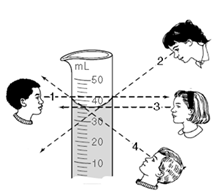# Physical Science Unit 1 Test - Scientific Investigation Quiz

30 Questions | Attempts: 124Settings• 1.
The process of making a conclusion from information provided is best described as a/an:
• A.

Control

• B.

Variable

• C.

Inference

• D.

Observation

• 2.
10m is equal to
• A.

100 cm

• B.

1,000 cm

• C.

11,000 mm

• 3.
For a hypothesis to be valid, it must be
• A.

Testable

• B.

Support by evidence

• C.

• D.

• 4.
A quantitative observation will include
• A.

Zoo animals

• B.

Numbers

• C.

Names

• 5.
The statement "Sheila has a stain on her shirt" is an example of a
• A.

Hypothesis

• B.

Qualitative observation

• C.

Prediction

• D.

Quantitative observation

• 6.
The use of the 5 senses to gather information is considered a/an
• A.

Observation

• B.

Inference

• C.

Prediction

• D.

Hypothesis

• 7.
When communicating experiment results in science your lab report should include:
• A.

Use tables and graphs if needed

• B.

Write a conclusion that states if your hypothesis was right or wrong

• C.

Make sure that it is free from bias

• D.

All of the above

• 8.
Scientists usually state an ______________________ in an "If ........then............" format.
• 9.
To describe the length of a grain of rice, you would use:
• A.

Millimeters (mm)

• B.

Decimeters (dm)

• C.

Meters (m)

• D.

Kilometers (km)

• 10.
Write the number of the position from which you should view the graduated cylinder to obtain the most accurate measurement.
• 11.
What is the mass of the rock in this picture?
• A.

350.50g

• B.

354.46g

• C.

534.46g

• D.

354.46ml

• 12.
The temperature on the thermometer shown below is:
• A.

21.5 °C.

• B.

22.5 °C.

• C.

25 °C.

• 13.
Physical Science is the study of two fields of science called Biology and Physics.
• A.

True

• B.

False

• 14.
Physical Science is the study of the relationship between matter and energy.
• A.

True

• B.

False

• 15.
What skills & methods are needed to understand scientific investigation?  Check all that apply.
• A.

Basic Science Skills

• B.

Scientific Method

• C.

Science Boot Camp

• 16.
What are some different ways to collect data & organize information in a useful way?  Check all that would apply.
• A.

Charts

• B.

Graphs

• C.

Scientific Notation

• D.

Ship it off to another lab

• E.

• 17.
What tool is used to measure the volume of an irregularly-shaped solid?
• A.

Ruler

• B.

• C.

Balance

• D.

Thermometer

• 18.
What does the pre-fix milli- mean?
• A.

.1

• B.

.001

• C.

100

• 19.
Which metric prefix means "thousand:?
• A.

Milli

• B.

Cento

• C.

Deka

• D.

Kilo

• 20.
We use the phrase "King Henry Doesn't Usually Drink Chocolate Milk" to remember the six most common SI units for measurement.
• A.

True

• B.

False

• 21.
The formula to find density is: (hint:  I love you)
• A.

Density = mass /volume

• B.

Density = volume / mass

• C.

Density = mass / vitamins

• 22.
The formula for finding the volume of a box to ship Mrs. Stevens wine from California is:
• A.

H x M x L

• B.

L x V x M

• C.

L x L x H

• D.

L x W x H

• 23.
A large marble is added to a graduated cylinder containing 80 mL of water, raising the level of the water to 90 mL.  What is the volume of the MARBLE in the graduated cylinder?
• A.

10 cm3

• B.

90 mL

• C.

80 cm3

• 24.
Which graph is best used to measure a LARGE rate of change over time?
• A.

Bar Graph

• B.

Circle Graph (pie chart)

• C.

Venn Diagram

• D.

Line Graph

• 25.
Mass and weight are the same thing.
• A.

True

• B.

False

## Related TopicsBack to top
×

Wait!
Here's an interesting quiz for you.